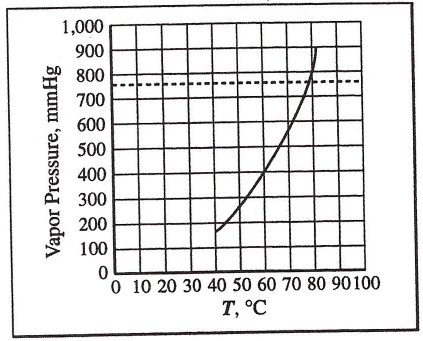# Problem: The graph shows how the vapor pressure of a liquid changes with temperature. Select the choice that best indicates the degree of correctness of this statement: "The normal boiling point of the liquid is 78°C."(A) The statement is true.(B) The statement is probably true; additional data would be needed for a final decision.(C) The statement is probably false; additional data would be needed for a final decision.(D) The statement is false.

###### FREE Expert Solution
81% (183 ratings)
###### Problem Details

The graph shows how the vapor pressure of a liquid changes with temperature. Select the choice that best indicates the degree of correctness of this statement: "The normal boiling point of the liquid is 78°C."

(A) The statement is true.

(B) The statement is probably true; additional data would be needed for a final decision.

(C) The statement is probably false; additional data would be needed for a final decision.

(D) The statement is false.What scientific concept do you need to know in order to solve this problem?

Our tutors have indicated that to solve this problem you will need to apply the Phase Diagram concept. You can view video lessons to learn Phase Diagram. Or if you need more Phase Diagram practice, you can also practice Phase Diagram practice problems.

What is the difficulty of this problem?

Our tutors rated the difficulty ofThe graph shows how the vapor pressure of a liquid changes w...as medium difficulty.

How long does this problem take to solve?

Our expert Chemistry tutor, Dasha took 2 minutes and 42 seconds to solve this problem. You can follow their steps in the video explanation above.

What professor is this problem relevant for?

Based on our data, we think this problem is relevant for Professor Hampton's class at UCF.